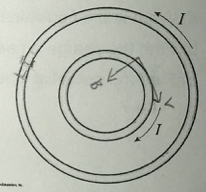# Problem: One long solenoid is placed inside another solenoid. Both solenoids have the same length and the same number of turns of wire, but the outer solenoid has twice the diameter of the inner solenoid. Each solenoid carries the same current, but the two currents are in opposite directions, as shown in the figure to the right. What is the magnetic field at the center of the inner solenoid?

###### FREE Expert Solution

Magnetic field due to solenoid:

$\overline{){\mathbf{B}}{\mathbf{=}}\frac{{\mathbf{\mu }}_{\mathbf{0}}\mathbf{iN}}{\mathbf{L}}}$

96% (14 ratings)###### Problem Details

One long solenoid is placed inside another solenoid. Both solenoids have the same length and the same number of turns of wire, but the outer solenoid has twice the diameter of the inner solenoid. Each solenoid carries the same current, but the two currents are in opposite directions, as shown in the figure to the right. What is the magnetic field at the center of the inner solenoid?